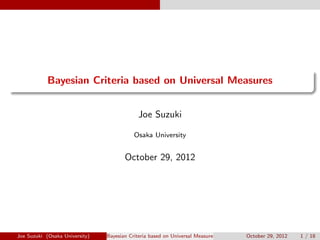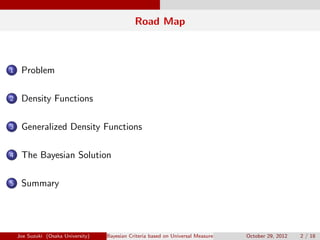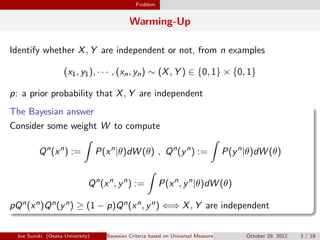Successfully reported this slideshow.

# Bayesian Criteria based on Universal Measures

1

Share×
1 of 18
1 of 18

# Bayesian Criteria based on Universal Measures

1

Share

Joe Suzuki, Bayesian Criteria based on Universal Measures, ISITA 2012, Hawaii, USA

Joe Suzuki, Bayesian Criteria based on Universal Measures, ISITA 2012, Hawaii, USA

## More Related Content

### Related Books

Free with a 14 day trial from Scribd

See all

### Related Audiobooks

Free with a 14 day trial from Scribd

See all

### Bayesian Criteria based on Universal Measures

1. 1. . ...... Bayesian Criteria based on Universal Measures Joe Suzuki Osaka University October 29, 2012 Joe Suzuki (Osaka University) Bayesian Criteria based on Universal Measures October 29, 2012 1 / 18
2. 2. Road Map ...1 Problem ...2 Density Functions ...3 Generalized Density Functions ...4 The Bayesian Solution ...5 Summary Joe Suzuki (Osaka University) Bayesian Criteria based on Universal Measures October 29, 2012 2 / 18
3. 3. Problem Warming-Up Identify whether X, Y are independent or not, from n examples (x1, y1), · · · , (xn, yn) ∼ (X, Y ) ∈ {0, 1} × {0, 1} p: a prior probability that X, Y are independent . The Bayesian answer .. ...... Consider some weight W to compute Qn (xn ) := ∫ P(xn |θ)dW (θ) , Qn (yn ) := ∫ P(yn |θ)dW (θ) Qn (xn , yn ) := ∫ P(xn , yn |θ)dW (θ) pQn(xn)Qn(yn) ≥ (1 − p)Qn(xn, yn) ⇐⇒ X, Y are independent Joe Suzuki (Osaka University) Bayesian Criteria based on Universal Measures October 29, 2012 3 / 18
4. 4. Problem Today’s Exercise A similar problem but what if (X, Y ) ∈ [0, 1) × {1, 2, · · · }. . Problem .. ......Construct something like Qn(xn), Qn(yn), Qn(xn, yn). Extend the idea without assuming either discrete or continuous Joe Suzuki (Osaka University) Bayesian Criteria based on Universal Measures October 29, 2012 4 / 18
5. 5. Problem What Qn is qualiﬁed to be an alternative to Pn ? θ∗: true θ   Pn(xn) = P(xn|θ∗), Pn(yn) = P(yn|θ∗) Pn(xn, yn) = Pn(xn, yn|θ)   Qn (xn ) := ∫ P(xn |θ)dW (θ) , Qn (yn ) := ∫ P(yn |θ)dW (θ) Qn (xn , yn ) := ∫ P(xn , yn |θ)dW (θ) Joe Suzuki (Osaka University) Bayesian Criteria based on Universal Measures October 29, 2012 5 / 18
6. 6. Problem Example: Bayes Codes c: the # of ones in xn P(xn |θ) = θc (1 − θ)n−c a > 0 w(θ) ∝ 1 θa(1 − θ)a   For each xn = (x1, · · · , xn) ∈ {0, 1}n, Qn (xn ) := ∫ w(θ)P(xn |θ)dθ Joe Suzuki (Osaka University) Bayesian Criteria based on Universal Measures October 29, 2012 6 / 18
7. 7. Problem Universal Coding/Measures If we choose a = 1/2 (Krichevsky-Troﬁmov) and xn is i.i.d. emitted by Pn (xn ) = n∏ i=1 P(xi ) then, for any P, almost surely, − 1 n log Qn (xn ) → H := ∑ x∈A −P(x) log P(x) From Shannon McMillian Breiman, for any P, − 1 n log Pn (xn ) = 1 n n∑ i=1 − log P(xi ) → E[− log P(xi )] = H Joe Suzuki (Osaka University) Bayesian Criteria based on Universal Measures October 29, 2012 7 / 18
8. 8. Problem The Essential Problem For any P, almost surely, 1 n log Pn(xn) Qn(xn) → 0 (1) (explains why Pn can be replaced by Qn if n is large) . X is neither discrete nor continuous .. ......What are Qn and (1) in the general settings ? Joe Suzuki (Osaka University) Bayesian Criteria based on Universal Measures October 29, 2012 8 / 18
9. 9. Density Functions Suppose a density function exists for X A: the range of X A0 := {A} Aj+1 is a reﬁnement of Aj Example 1: if A0 = {[0, 1)}, the sequence can be A1 = {[0, 1/2), [1/2, 1)} A2 = {[0, 1/4), [1/4, 1/2), [1/2, 3/4), [3/4, 1)} . . . Aj = {[0, 2−(j−1)), [2−(j−1), 2 · 2−(j−1)), · · · , [(2j−1 − 1)2−(j−1), 1)} . . . sj : A → Aj (projection, x ∈ a ∈ Aj =⇒ sj (x) = a) λ : R → B (Lebesgue measure, a = [b, c) =⇒ λ(a) = c − b) Joe Suzuki (Osaka University) Bayesian Criteria based on Universal Measures October 29, 2012 9 / 18
10. 10. Density Functions If (sj (x1), · · · , sj (xn)) = (a1, · · · , an), gn j (xn ) := Qn j (a1, · · · , an) λ(a1) · · · λ(an) f n j (xn ) := fj (x1) · · · fj (xn) = Pj (a1) · · · Pj (an) λ(a1) . . . λ(an) For {ωj }∞ j=1: ∑ ωj = 1, ωj > 0, gn (xn ) := ∞∑ j=1 ωj gn j (xn ) If we choose {Ak} such that fk → f , for any f , almost surely 1 n log f n(xn) gn(xn) → 0 (2) B. Ryabko. IEEE Trans. on Inform. Theory, 55, 9, 2009. Joe Suzuki (Osaka University) Bayesian Criteria based on Universal Measures October 29, 2012 10 / 18
11. 11. Generalized Density Functions Exactly when does density function exist? B: the Borel sets of R µ(D): the probabbility of D ∈ B . When a density function exists .. ...... The following are equivalent (µ ≪ λ): for each D ∈ B, λ(D) = 0 =⇒ µ(D) = 0 ∃ B-measurable dµ dλ := f s.t. µ(D) = ∫ D f (t)dλ(t) Joe Suzuki (Osaka University) Bayesian Criteria based on Universal Measures October 29, 2012 11 / 18
12. 12. Generalized Density Functions Density Functions in a General Sense . Radon-Nikodum’s Theorem .. ...... The following are equivalent (µ ≪ η): for each D ∈ B, η(D) = 0 =⇒ µ(D) = 0 ∃ B-measurable dµ dη := f s.t. µ(D) = ∫ D f (t)dη(t) Example 2: µ({k}) > 0, η({j}) := 1 k(k + 1) , k ∈ B := {1, 2, · · · } µ ≪ η µ(D) = ∑ k∈D∩B f (k)η({k}) dµ dη (k) = f (k) = µ({k}) η({k}) = k(k + 1)µ({k}) Joe Suzuki (Osaka University) Bayesian Criteria based on Universal Measures October 29, 2012 12 / 18
13. 13. Generalized Density Functions In this work, ... B1 := {{1}, {2, 3, · · · }} B2 := {{1}, {2}, {3, 4, · · · }} . . . Bk := {{1}, {2}, · · · , {k}, {k + 1, k + 2, · · · }} . . . tk : B → Bk (projection, y ∈ b ∈ Bk =⇒ tk(y) = b) If (tk(y1), · · · , tk(yn)) = (b1, · · · , bn), gn k (yn ) := Qn k (b1, · · · , bn) η(b1) · · · η(bn) , gn (yn ) := ∞∑ k=1 ωkgn k (yn ) If we choose {Bk} s.t. fk → f , for any f , almost surely 1 n log f n(yn) gn(yn) → 0 (3) gn(yn) ∏n i=1 ηn({yi }) estimates P(yn) = f n(yn) ∏n i=1 ηn({yi }) Joe Suzuki (Osaka University) Bayesian Criteria based on Universal Measures October 29, 2012 13 / 18
14. 14. Generalized Density Functions Joint Density Functions Example 3: A × B (based on Examples 1,2) µ ≪ λη A0 × B0 = {A} × {B} = {[0, 1)} × {{1, 2, · · · }} A1 × B1 A2 × B2 . . . Aj × Bk . . . (sj , tk) : A × B → Aj × Bk   If {Aj × Bk} satisﬁes fjk → f , for any f , almost surely, we can construct gn s.t. 1 n log f n(xn, yn) gn(xn, yn) → 0 (4) Joe Suzuki (Osaka University) Bayesian Criteria based on Universal Measures October 29, 2012 14 / 18
15. 15. The Bayesian Solution The Answer to Today’s Problem Estimate f n X (xn), f n Y (yn), f n XY (xn, yn) by gn X (xn), gn Y (yn), gn XY (xn, yn)   . The Bayesian answer .. ......pgn X (xn)gn Y (yn) ≤ (1 − p)gXY (xn, yn) ⇐⇒ X, Y are independent Joe Suzuki (Osaka University) Bayesian Criteria based on Universal Measures October 29, 2012 15 / 18
16. 16. The Bayesian Solution The General Bayesian Solution Givem n example zn and prior {pm} over models m = 1, 2, · · · , compute gn(zn|m) for each m = 1, 2, · · · ﬁnd the model m maxmizing pmg(zn|m) Joe Suzuki (Osaka University) Bayesian Criteria based on Universal Measures October 29, 2012 16 / 18
17. 17. The Bayesian Solution Universality in the generalized sense 1 n log f n(zn) gn(zn) → 0 µn (Dn ) := ∫ D f n (zn )dηn (zn ) νn (Dn ) := ∫ D gn (zn )dηn (zn ) f n(zn) gn(zn) = dµn dηn (zn )/ dνn dηn (zn ) = dµn dνn (zn ) . Universality .. ...... 1 n log dµn dνn (zn ) → 0 Joe Suzuki (Osaka University) Bayesian Criteria based on Universal Measures October 29, 2012 17 / 18
18. 18. Summary Summary and Discussion . Bayesian Measure .. ...... Generalization without assuming Discrete or Continuous Universality of Bayes/MDL in the generalized sense . Many Applications .. ...... Bayesian network structure estimation (DCC 2012) The Bayesian Chow-Liu Algorithm (PGM 2012) Markov order estimation even when {Xi } is continuous Joe Suzuki (Osaka University) Bayesian Criteria based on Universal Measures October 29, 2012 18 / 18